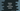# Python program to print the harmonic series## Python program to print the harmonic series

In this post, we will learn different ways to print the harmonic series in Python. Harmonic series is a series of numbers, the reciprocal of which form an arithmetic series.

For example, 1, 1/2, 1/3, 1/4… is a harmonic series. If we take the reverse of each number, we will get the arithmetic series 1, 2, 3, 4….

We can use a loop to print a harmonic series in Python. We can print the actual number values or we can print these as strings.

### Algorithm:

Before we start writing the program, let’s understand how the algorithm works. The reciprocal of the items of a harmonic series creates an arithmetic progression.

The nth item of an arithmetic progression is a + (n - 1) * d. Where,

• a is the first number of the series.
• d is the common difference.

So, the nth item of a harmonic progression is 1/(a + (n - 1) * d)

In the loop, we will use this formula to find the nth term and print it to the user.

### Method 1: Python program to print the number values of a harmonic progression:

The below example uses a for loop to print the harmonic progression. It prints the number values:

``````n = int(input("Enter the value of n: "))
a = int(input("Enter the value of a: "))
d = int(input("Enter the value of d: "))

for i in range(1, n+1):
v = 1/(a + (i - 1) * d)
print(v, end=" ")``````

For this example, we are taking the values of a, n and d as inputs from the user. The for loop runs from 1 to n. On each iteration of this loop, we are calculating the nth value. Here, we have to calculate the ith value of the harmonic series.

If you run this program, it will give output as like below:

``````Enter the value of n: 5
Enter the value of a: 1
Enter the value of d: 1
1.0 0.5 0.3333333333333333 0.25 0.2``````

### Method 2: Python program to print the harmonic progression as string values:

We can also print the values as strings. Instead of printing the number values, it will print the values as 1, 1/2, 1/3, 1/4….

Let’s change the program to print the series with string values:

``````n = int(input("Enter the value of n: "))
a = int(input("Enter the value of a: "))
d = int(input("Enter the value of d: "))

for i in range(1, n+1):
v = a + (i - 1) * d

if v == 1:
print("1", end=" ")
else:
print(f'1/{v}', end=" ")``````

Here, v is the denominator. If it is equal to 1, it prints 1, else it prints 1/v.

If you run this program, it will print output as like below:

``````Enter the value of n: 5
Enter the value of a: 1
Enter the value of d: 1
1 1/2 1/3 1/4 1/5

Enter the value of n: 10
Enter the value of a: 2
Enter the value of d: 2
1/2 1/4 1/6 1/8 1/10 1/12 1/14 1/16 1/18 1/20``````

### Method 3: Python program to print the harmonic series with a while loop:

Similar to a for loop, we can also use a while loop to print the harmonic series. Below program uses a while loop to print the series:

``````n = int(input("Enter the value of n: "))
a = int(input("Enter the value of a: "))
d = int(input("Enter the value of d: "))

i = 1
while i < n+1:
v = 1/(a + (i - 1) * d)
print(v, end=" ")
i = i + 1``````

We are initializing i as 1 before the loop starts and its value is incremented by 1 after each iteration. It will print similar output.